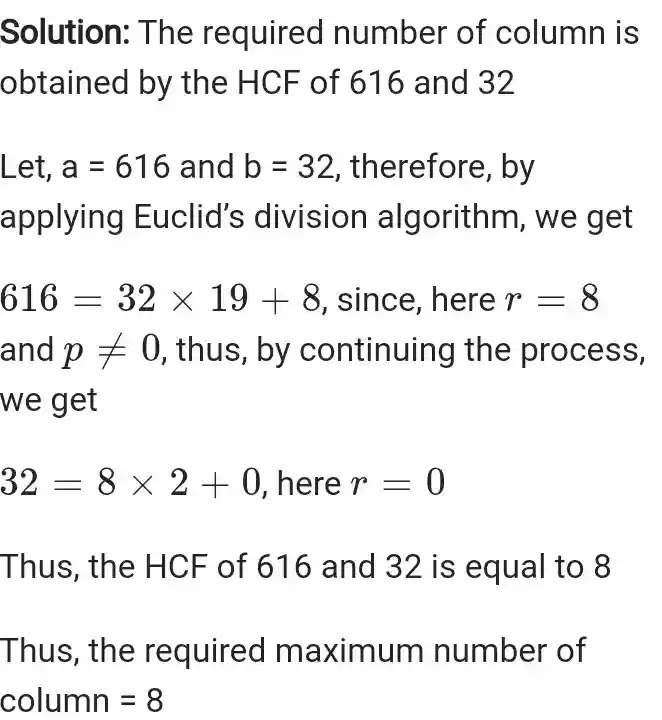# NCERT Solutions for Class 10 Maths Exercise 1.1

NCERT Solutions for Class 10 Maths Chapter 1 Real Numbers Exercise 1.1
NCERT Solutions for Class 10 Maths Chapter 1 Real Numbers Ex 1.1 are part of NCERT Solutions for Class 10 Maths. Here we have given NCERT Solutions for Class 10 Maths Chapter 1 Real Number Exercise 1.1

## NCERT Solutions for Class 10 Maths Exercise 1.1

Ex 1.1 Class 10 Maths Question 1.
Use Euclid’s Division Algorithm to find the HCF of:

Ex 1.1 Class 10 Maths Question 3.
An army contingent of 616 members is to march behind an army band of 32 members in a parade. The two groups are to march in the same number of columns. What is the maximum number of columns in which they can marchEx 1.1 Class 10 Maths Question 4.

### NCERT Solutions for Class 10 Maths Chapter 1 Real Numbers Exercises

Exercise 1.1 of NCERT solutions for class 10 maths chapter 1 Real Numbers is the first exercise of Chapter 1 of Class 10 Maths. Real Numbers is introduced in Class 9 and this is discussed further in detail in Class 10 by studying Euclid’s division Algorithm. The exercise discusses the divisibility of integers. The divisibility of integers using Euclid’s division algorithm says that any positive integer a can be divided by another positive integer b such that the remainder will be which is smaller than b.NCERT Solutions, NCERT Solutions Class 10, NCERT Solutions Class 10 Maths, NCERT Solutions Class 10 Maths Chapter 1, NCERT Solutions Class 10 Maths Chapter 1 Real Numbers, NCERT Solutions Class 10 Maths Chapter 1 Real Numbers Exercise 1.1, NCERT Solutions Class 10 Maths Chapter 1 Exercise 1.1, NCERT Solutions Class 10 Maths Exercise 1.1, Class 10 Maths Chapter 1 Real Numbers Exercise 1.1, Class 10 Maths Chapter 1 Exercise 1.1, Class 10 Maths Chapter 1 Real Numbers Exercise 1.1, Exercise 1.1 Class 10 Maths Solution, Class 10 Maths Exercise 1.1 Solution with PDF, Class 10 Maths Chapter 1 Exercise 1.1 with PDF, Class 10 Maths Exercise 1.1 Solution, Ex. 1.1 Class 10 Maths, NCERT Solutions Class 10 Maths Chapter 1 PDF, NCERT Solutions Class 10 Exercise 1.1, Class 10 Maths Solution PDF, Class 10 Maths